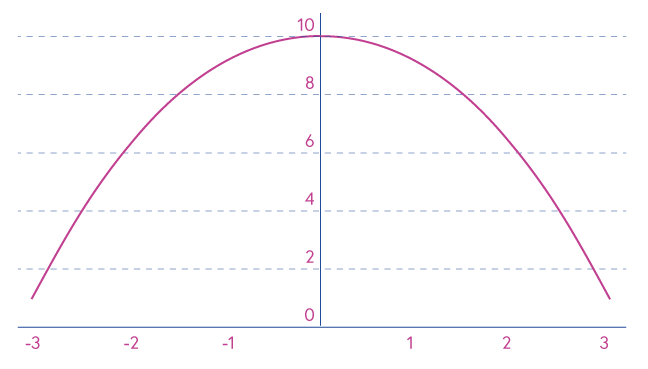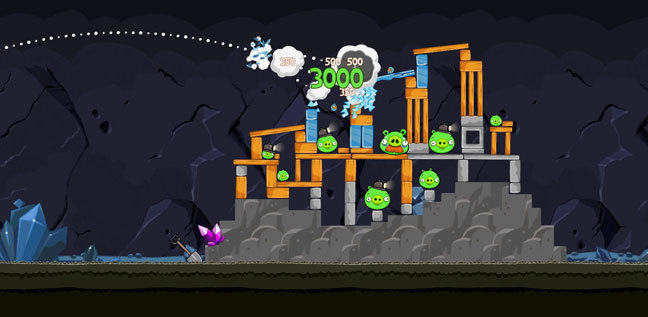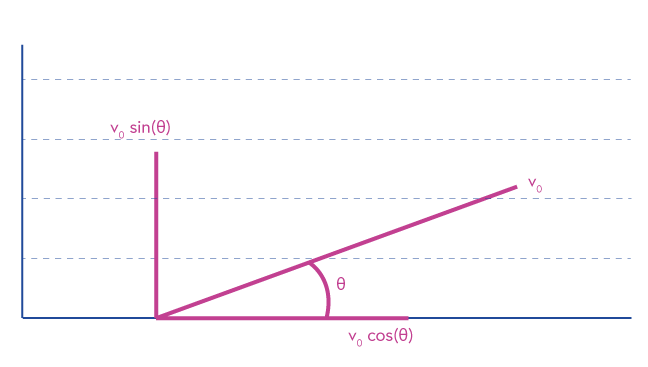## Want to keep learning?

This content is taken from the University of Leicester's online course, Real World Calculus: How Maths Drives Formula One and Launches Angry Birds. Join the course to learn more.
2.10

# Projectile motion

In this section we will discuss projectile motion, or how calculus can be used to launch Angry Birds.

When launched, projectiles fly in a parabolic path. If you haven’t come across this word before it just means the shape of the graph of $$10–x^2$$ as shown here:Note that in this example it is only the shape of the graph which is important. We have added the +10 simply so that the curve lies above the x-axis

This sort of curve should look familiar if you watched the Angry Birds video in the previous activity.

When we consider a projectile moving through the air we will assume that there is no air resistance. While this is not always a good assumption to make on Earth, it does allow us to understand the flight of the birds in the well-known Angry Birds game and means we can easily switch our thinking to motion in space, where there is no air at all. In fact we will assume that there are no horizontal forces acting upon the projectile and we will only consider the effect of gravity acting on our projectile.© Rovio Entertainment Ltd

In the case of our simple projectile, there are only two parameters that affect its trajectory: the initial velocity and the launch angle. When we launch an Angry Bird we have to do two things, aim (pick the angle) and decide how much power to use (pick the initial velocity). If we call the initial velocity $$v_0$$ and the angle at which the projectile is launched $$\theta$$ then we can consider the vertical and horizontal components of the velocity and acceleration separately.

In order to do this we will need to use some trigonometry. We will not attempt to explain it in full, but if you are comfortable with trigonometry and you want to understand the full explanation you can find it here.

For our purposes, we simply need to know that we can describe the speed and the acceleration of a projectile in terms of components of these quantities that are directed horizontally (along the ground) and vertically.

The Greek letter theta ($$\theta$$) is used to represent the angle at which the projectile is launched.

Taking this into account we have the following variables:
The initial velocity of projectile: $$v_0$$
The angle at which projectile is launched: $$\theta$$
The initial velocity in horizontal direction: $$v_0 \cos(\theta)$$
The initial velocity in vertical direction: $$v_0 \sin(\theta)$$The acceleration in horizontal direction: 0
The acceleration in vertical direction: -9.8 m $$s^{-2}$$

The -9.8 figure is due to gravity and we will explore it in the next Step.